https://doi.org/10.5194/acp-23-14115-2023
https://doi.org/10.5194/acp-23-14115-2023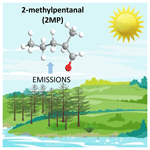# Atmospheric impact of 2-methylpentanal emissions: kinetics, photochemistry, and formation of secondary pollutants

María Asensio, Sergio Blázquez, María Antiñolo, José Albaladejo, and Elena Jiménez
Abstract

The tropospheric fate of 2-methylpentanal (2MP) has been investigated in this work. First, the photochemistry of 2MP under simulated solar conditions was investigated by determining the UV absorption cross sections (220–360 nm) and the effective photolysis quantum yield in the UV solar actinic region (λ> 290 nm). The photolysis rate coefficient in that region was estimated using a radiative transfer model. Photolysis products were identified by Fourier transform infrared (FTIR) spectroscopy. Secondly, a kinetic study of the chlorine (Cl) and hydroxyl (OH) reactions of 2MP was also performed at 298 K and as a function of temperature (263–353 K), respectively. For the Cl reaction, a relative kinetic method was used in a smog chamber coupled to FTIR spectroscopy, whereas for the OH reaction, the pulsed laser photolysis (PLP) with laser-induced fluorescence (LIF) technique was employed. The estimated lifetime of 2MP depends on the location, the season, and the time of the day. Under mild–strong irradiation conditions, UV photolysis of 2MP may compete with its OH reaction in a mid-latitude inland urban atmosphere, while Cl reaction dominates in mid-latitude coastal urban areas at dawn. Finally, the gaseous product distribution of the Cl and OH reactions was measured in a smog chamber as well as the formation of secondary organic aerosols (SOAs) in the Cl reaction and its size distribution (diameter between 5.6 and 560 nm). The implications for air quality are discussed based on the observed products.

Share
Dates
1 Introduction

The World Health Organization (WHO) estimates that air pollution is responsible for around 7 million deaths worldwide. One of the damaging air pollutants is particulate matter (PM). Concretely, fine and ultrafine particles (UFPs), with diameters smaller than 2.5 and 0.1 µm, respectively, are extremely dangerous since they penetrate deep into the lungs and enter the bloodstream. UFPs and other secondary pollutants are formed in the gas-phase reactions of volatile organic compounds (VOCs), such as aldehydes, with oxidants such as hydroxyl (OH) radicals or chlorine (Cl) atoms. In the atmosphere, OH radicals are ubiquitous, while Cl is mainly present in marine or coastal atmospheres since it is formed from reactions of sea-salt aerosols (Atkinson et al., 1995; Wennberg et al., 2018; Rodríguez et al., 2012; Atkinson and Arey, 2003; Antiñolo et al., 2019, 2020). Globally, ceramic industries and coal-fired power plants are relevant sources of Cl2 inland (Galán et al., 2002; Sarwar and Bhave, 2007; Deng et al., 2014) and, therefore, of Cl atoms.

Saturated aldehydes, like formaldehyde, acetaldehyde, pentanal, and hexanal, are emitted into the atmosphere (Clarisse et al., 2003; Calvert et al., 2011; Villanueva et al., 2022) mainly from primary sources, e.g., natural gas from power stations, landfill gas, flaring from offshore, and transport (Calvert et al., 2011). But they can also be formed in situ in the atmosphere as secondary pollutants from reactions of alkenes and alcohols with oxidants (Atkinson et al., 1995; Wennberg et al., 2018; Rodríguez et al., 2012; Atkinson and Arey, 2003). In this work, we focus on the diurnal atmospheric chemistry of 2-methylpentanal (2MP, CH3CH2CH2CH(CH3)C(O)H) and its impact in the troposphere in terms of its lifetime and generated products. This aldehyde has been detected in the atmosphere (Xu et al., 2003) as it can be released to the environment from some foods (Aisala et al., 2019) and from contaminated water in waste streams (Bao et al., 1998), since it is widely used as a flavoring ingredient and as an intermediate in the synthesis of dyes, resins, and pharmaceuticals (Furia and Bellanca, 1975). Moreover, 2MP has been detected in ambient air at the foot of Mount Everest (Ciccioli et al., 1993). The former source of 2MP implies that it comes from localized sources, but the latter one could imply that 2MP is both long-lived and well-mixed throughout the troposphere or has a specific local source in that remote region. In addition, 2MP has been detected in certain indoor environments from emission of cigarette smoke (Lippmann, 2000).

Once emitted, 2MP can be degraded during the daytime by different processes forming secondary pollutants (gases and/or PM) that can have a significant impact on air quality and health. In the atmosphere, these processes include ultraviolet (UV) photolysis by the solar actinic radiation (Reaction R1), reaction with Cl atoms (Reaction R2), and/or reaction with OH radicals (Reaction R3),

$\begin{array}{}\text{(R1)}& \begin{array}{rl}{\mathrm{CH}}_{\mathrm{3}}& {\mathrm{CH}}_{\mathrm{2}}{\mathrm{CH}}_{\mathrm{2}}\mathrm{CH}\left({\mathrm{CH}}_{\mathrm{3}}\right)\mathrm{C}\left(\mathrm{O}\right)\mathrm{H}+h\mathit{\nu }\left(\mathit{\lambda }\ge \mathrm{290}\phantom{\rule{0.125em}{0ex}}\mathrm{nm}\right)\\ & \to \mathrm{products}\phantom{\rule{2em}{0ex}}J,\end{array}\text{(R2)}& \begin{array}{rl}{\mathrm{CH}}_{\mathrm{3}}& {\mathrm{CH}}_{\mathrm{2}}{\mathrm{CH}}_{\mathrm{2}}\mathrm{CH}\left({\mathrm{CH}}_{\mathrm{3}}\right)\mathrm{C}\left(\mathrm{O}\right)\mathrm{H}+\mathrm{Cl}\\ & \to \mathrm{products}\phantom{\rule{2em}{0ex}}{k}_{\mathrm{Cl}},\end{array}\text{(R3)}& \begin{array}{rl}{\mathrm{CH}}_{\mathrm{3}}& {\mathrm{CH}}_{\mathrm{2}}{\mathrm{CH}}_{\mathrm{2}}\mathrm{CH}\left({\mathrm{CH}}_{\mathrm{3}}\right)\mathrm{C}\left(\mathrm{O}\right)\mathrm{H}+\mathrm{OH}\\ & \to \mathrm{products}\phantom{\rule{2em}{0ex}}{k}_{\mathrm{OH}}.\end{array}\end{array}$

To our knowledge, the photolysis rate coefficient (J) of 2MP in the atmosphere and the rate coefficient for Reaction (R2), kCl, have not been reported to date in the literature. D'Anna et al. (2001) reported the rate coefficient for the 2MP + OH reaction (Reaction R3), kOH, at 298 K and 760 Torr of air. The reaction products of Reactions (R1)–(R3) are still unknown.

In this work, the evaluation of the atmospheric fate of 2MP has been carried out under NOx-free conditions, simulating a clean atmosphere. Therefore, the UV photodissociation of 2MP has been investigated at room temperature, determining the UV absorption cross sections (σλ) between 220 and 360 nm and the effective photolysis quantum yield (Φeff) and J at an altitude z and a solar zenith angle θ in the actinic region in a Spanish mid-latitude inland city (Ciudad Real) and a coastal city (Valencia). The rate coefficients of Reactions (R2) and (R3) have been determined by the relative method using Fourier transform infrared (FTIR) spectroscopy and by pulsed laser photolysis–laser-induced fluorescence (PLP–LIF), respectively. The gas-phase products of Reactions (R1)–(R3) have been also detected by gas chromatography (GC) coupled to mass spectrometry (MS), FTIR spectroscopy, and proton transfer reaction time-of-flight mass spectrometry (PTR-ToF-MS). No information on the formation of secondary organic aerosols (SOAs) of Reactions (R1)–(R3) has been reported yet. In this work, we study the SOA formation in Reaction (R2) by using a fast-mobility particle sizer spectrometer (FMPS). The potential implications of the diurnal degradation processes of 2MP are discussed in terms of the estimated lifetime in mid-latitude inland and coastal urban atmospheres. The identification of the gaseous products formed in Reactions (R1)–(R3) as well as the formation of submicron particles in the Reaction (R2) provides a better understanding of the tropospheric photochemistry of 2MP and its impact on air quality.

2 Experimental section

## 2.1 Atmospheric photodissociation of 2MP

The experimental systems and procedures have been described in detail elsewhere (Blázquez et al., 2020; Asensio et al., 2022), so only a brief description is given here. The UV absorption cross sections of 2MP (σλ in base e) were determined by UV absorption spectroscopy (Blázquez et al., 2020) between 220 and 360 nm at (298±1) K. A cylindrical cell with an optical pathlength of 107.15 cm was filled with gas-phase 2MP in the range of 1.02–9.65 Torr and irradiated by a deuterium lamp (DT-200, StellarNet). The transmitted radiation was detected by a 2048-pixel CCD camera coupled to a Czerny–Turner spectrograph (BLACK-Comet model C, StellarNet) with 3 nm spectral resolution. σλ values were determined from the slopes of Beer–Lambert's law plots, such as shown in Fig. S1 and as described in the Supplement.

The experimental setup used to investigate the photolysis of 2MP by sunlight under atmospheric conditions ((298±1) K and (760±2) Torr of air) has been described elsewhere (Asensio et al., 2022). Briefly, a 20 cm long cylindrical jacketed cell was filled with diluted 2MP in synthetic air (dilution factors, P2MP$/$ (P2MP+Pair), ranged from $\mathrm{1.07}×{\mathrm{10}}^{-\mathrm{3}}$ to $\mathrm{1.73}×{\mathrm{10}}^{-\mathrm{3}}$). The temperature of the gas mixture in the cell was kept constant by recirculating water through the jacket from a thermostatic bath (CD-200F, CORIO). The gas mixture was irradiated with a solar simulator (model 11002-2, SunLite™) at λ> 290 nm for 30, 60, 90, 120, or 150 min. Average irradiance was (2.335±0.126) suns. One sun is the irradiance of the AM1.5G reference solar spectrum (Gueymard et al., 2002). At each photolysis time, the gas mixture (unreacted 2MP and reaction products) was transferred to a 16 L white-type cell, with an optical path length of 71 m, coupled to an FTIR spectrometer (Nicolet Nexus 870, Thermo Fisher Scientific) (Asensio et al., 2022) with a 2 cm−1 resolution. Spectra were recorded between 650 and 4000 cm−1 and after the accumulation of 32 interferograms. The selected IR bands for monitoring 2MP were those centered at 1748 cm−1and between 2630 and 3000 cm−1.

In some experiments, cyclohexane was added to the gas mixture as a radical scavenger ([cyclohexane]0$/$ [2MP]0 = 8.8–10.5) to evaluate the impact of secondary chemistry. Out of a total of 10 experiments, 6 experiments were performed adding cyclohexane, finding no difference in the photolysis rate coefficient, J, and photoproducts were identified in the absence of cyclohexane. In addition, dark experiments were performed to evaluate the loss of 2MP by heterogeneous reaction onto the reactor walls. The rate coefficient, kheterog, was determined to be $\mathrm{1.43}×{\mathrm{10}}^{-\mathrm{5}}$ s−1. The heterogeneous loss process accounts for 39 % of the total 2MP loss. Therefore, the total loss of 2MP after irradiation was corrected with kheterog to obtain J (in s−1) from the slope of the plot of ln([2MP]0$/$ [2MP]t) versus time according to Eq. (1):

$\begin{array}{}\text{(1)}& \mathrm{ln}\left(\left[\mathrm{2}\mathrm{MP}{\right]}_{\mathrm{0}}/\left[\mathrm{2}\mathrm{MP}{\right]}_{t}\right)=\left({k}_{\mathrm{heterog}}+J\right)t,\end{array}$

where the subscripts 0 and t refer to the concentrations of 2MP at initial time and elapsed time t, respectively. The initial concentration of 2MP in the photolysis cell ranged from 1.12×1016 to 6.55×1016 molecules cm−3 (i.e., 455–2663 ppm).

## 2.2 Gas-phase kinetics of the reaction of 2MP with Cl and OH

### 2.2.1 Relative measurements of kCl

The relative rate methodology and the experimental system to determine kCl at (298±2) K and (760±5) Torr have already been described (Antiñolo et al., 2019, 2020). In this work, isoprene and propene were used as reference compounds which react with Cl (kref) in competition with 2MP. The mixture of 2MP, Cl2, one of the reference compounds, and synthetic air was introduced into the 16 L cell described above, and FTIR spectroscopy was used as a detection technique to monitor 2MP and the reference compounds (isoprene or propene). IR spectra were recorded every 2 min, and the IR bands selected for monitoring 2MP, isoprene, and propene were 2630–3000, 3100, and 3050–3100 cm−1, respectively. Ranges of initial concentrations were [2MP]0= (3.7–9.4) × 1014 molecules cm−3 (15–38 ppm), [isoprene]0= (3.7–6.2) × 1014 molecules cm−3 (15–25 ppm) or [propene]0= (4.2–6.2) × 1014 molecules cm−3 (17–25 ppm), and [Cl2]0= (3.4–4.2) ×1014 molecules cm−3 (14–17 ppm). Cl atoms were generated in situ by photolysis of Cl2 by three actinic lamps (Philips Actinic BL TL 40W/10 1SL/25, λ= 340–400 nm) surrounding the cell. Both 2MP and the reference compound mainly react with Cl, but they can also be removed by heterogeneous reaction onto the reactor walls, UV photolysis, and/or reaction with Cl2. The rate coefficient for these three loss processes of 2MP (kloss) and the reference compound (kref,loss) was evaluated prior to each experiment (Antiñolo et al., 2019, 2020). As shown in Table S1, 2MP and the reference compounds only exhibited wall losses and no photolysis at the emission wavelengths of the actinic lamps was observed for any of them. Only isoprene reacts with Cl2.

Considering all the processes, the integrated rate equation is given by Eq. (2):

$\begin{array}{}\text{(2)}& \mathrm{ln}\left(\frac{\left[\mathrm{2}\mathrm{MP}{\right]}_{\mathrm{0}}}{\left[\mathrm{2}\mathrm{MP}{\right]}_{t}}\right)-{k}_{\mathrm{loss}}t=\frac{{k}_{\mathrm{Cl}}}{{k}_{\mathrm{ref}}}\left[\mathrm{ln}\left(\frac{\left[\mathrm{Ref}{\right]}_{\mathrm{0}}}{\left[\mathrm{Ref}{\right]}_{t}}\right)-{k}_{\mathrm{ref},\mathrm{loss}}t\right].\end{array}$

The total loss of 2MP and the reference compounds in the absence of Cl atoms was on the order of 10−5 s−1 in all cases.

### 2.2.2 Absolute measurements of kOH(T)

The pulsed laser photolysis–laser-induced fluorescence (PLP–LIF) technique was employed to determine kOH as a function of temperature (T= 263–353 K), kOH(T), and total pressure (PT= 50–500 Torr). The experimental setup was previously described (Martínez et al., 1999; Albaladejo et al., 2002; Jiménez et al., 2005; Antiñolo et al., 2012; Blázquez et al., 2017; Asensio et al., 2022); thereby a brief description is given here. The reactor consisted of a jacketed Pyrex cell (ca. 200 mL) through which a gas mixture formed by He (bath gas, main flow), H2O2$/$ He, and diluted 2MP is flown. The mass flow rates employed in this work are summarized in Table S2 of the Supplement.

The OH radicals were generated in situ by the PLP of gaseous H2O2 at 248 nm, radiation emitted by a KrF excimer laser (Coherent, Excistar 200). The OH radicals generated were subsequently excited at ca. 282 nm by doubling the output radiation of a Rhodamine-6G dye laser (LiopTec, LiopStar) pumped by the second harmonic of a Nd:YAG laser (InnoLas, SpitLight 1200). At 90 from photolysis and excitation lasers, the LIF at ca. 310 nm was collected by a filtered phototube (Thorn EMI, 9813B). At a constant T and PT, the pseudo-first-order rate coefficient, k, was obtained from the analysis of the LIF intensity decays shown in Fig. S2, at several initial concentrations of 2MP, [2MP]0= (0.30–5.91) ×1014 molecules cm−3. Then, kOH(T) was determined from the slope of k versus [2MP]0 plots. See the Supplement for more details.

## 2.3 Product studies of 2MP reactions with Cl (Reaction R2) and OH (Reaction R3)

### 2.3.1 Gaseous products

Briefly, two atmospheric simulation chambers were used to detect and identify the products generated in Reactions (R2) and (R3) at (759±3) Torr of air and (298±2) K: the 16 L cell described above coupled to the FTIR spectrometer (only used for the Cl reaction) (Ballesteros et al., 2017; Antiñolo et al., 2019) and a 264 L chamber (Antiñolo et al., 2020) from which the sample is injected into a GC–MS instrument (Thermo Electron, models Trace GC Ultra and DSQ II) (only used for the Cl reaction) (Asensio et al., 2022) or a PTR-ToF-MS (PTR TOF 4000, Ionicon). The 264 L chamber was surrounded by eight actinic lamps (Philips Actinic BL TL 40W/10 1SL/25, λ= 340–400 nm) to generate Cl atoms by Cl2 photolysis and four germicidal lamps (Philips TUV 36W SLV/6, λ= 254 nm) to generate OH radicals by H2O2 photolysis. In all cases, preliminary tests were carried out in the dark to check if products were generated in the potential reaction of 2MP with the oxidant precursor. In addition, in the absence of oxidant precursor, the formation of products during the UV light exposure of 2MP was also checked. For the product study of the OH reaction, 2MP $/$ H2O2$/$ air mixtures were irradiated for 70 min by the germicidal lamps, whereas in the Cl atoms experiments, 2MP $/$ Cl2$/$ air mixtures were irradiated with the actinic lamps for 60 min. IR spectra, chromatograms, and mass spectra were recorded every 2 min, 15 min, and 20 s, respectively.

The GC–MS instrument was equipped with a BPX35 column (30 m × 0.25 mm i.d. × 0.25 µm, SGE Analytical Science), and the solid-phase microextraction technique was used as a sampling method with a $\mathrm{50}/\mathrm{30}$µm divinylbenzene–carboxen–polydimethylsiloxane (DVB–CAR–PDMS) fiber (Supelco) exposed during 10 min to the gas mixture in the chamber. In these experiments, ranges for the initial concentrations were [2MP]0= (1.5–4.4) ×1014 molecules cm−3 (6–18 ppm) and [Cl2]0= (1.1–2.5) ×1014 molecules cm−3 (4–10 ppm). Note that the limit of detection (LOD) of the GC–MS instrument is 1×1011 molecules cm−3 (4 ppb).

In the FTIR experiments, initial concentrations were slightly different and were [2MP]0= (6.1–8.5) × 1014 molecules cm−3 (25–35 ppm) and [Cl2]0= (1.6–5.4) × 1014 molecules cm−3 (6–22 ppm) (LOD = 8 × 1011 molecules cm−3 (32 ppb)), while in the PTR-ToF-MS experiments (LOD = 1.2 × 108 molecules cm−3 (5 ppt)), [2MP]0= (1.3–2.3) × 1013 molecules cm−3 (0.5–0.9 ppm) and [Cl2]0= (1.1–1.4) × 1013 molecules cm−3 (0.4–0.6 ppm), which were lower than those used in the experiments using GC–MS instrument and FTIR due to the higher sensitivity of the PTR-ToF-MS. For the OH reaction monitored by PTR-ToF-MS, [H2O2]0= 3.8 × 1014 molecules cm−3 (15 ppm). The mass spectra of the reactive gas mixture were recorded with a time resolution of 20 s, and the detected mass range was set between 29 and 390.86 amu (atomic mass units) working at $E/N=$ 138 Td and Vdrift= 650 V. Prior to the in situ measurements, a calibration of the PTR-ToF-MS was made between 0.3×1013 and 2.9 × 1013 molecules cm−3 (122–1179 ppb).

Regarding the oxidant concentrations, [Cl] and [OH] were estimated from the first-order loss rate of 2MP measured by FTIR and PTR-ToF-MS. From FTIR experiments, [Cl] was estimated to range between 2.0×106 and 3.1 ×  106 atoms cm−3 (0.08–0.13 ppt), while in the PTR-ToF-MS experiments the estimated [Cl] was found to vary between 1.0×107 and 2.2 × 107 atoms cm−3 (0.4–0.9 ppt) and [OH] = 4.8 × 105 radicals cm−3 (0.02 ppt).

### 2.3.2 Secondary organic aerosols (SOAs)

To detect and quantify the SOA formation in the Cl reaction, the 264 L chamber, and the 16 L cell were connected in series, as described previously (Antiñolo et al., 2020). SOA experiments were performed in the absence of the reference compounds used in the relative kinetic study (isoprene or propene) to avoid any interference from their degradation initiated by Cl atoms. No seed was added to the gas mixture either. The concentration of 2MP was monitored by the FTIR spectrometer every 2 min, whereas the formed SOAs were monitored by a FMPS spectrometer (TSI 3091) every 1 min. Initial concentrations were 8.3 × 1014 molecules cm−3 (34 ppm) of 2MP and 7.8 × 1014 molecules cm−3 (32 ppm) of Cl2 ([Cl] was estimated to be 8.0 × 105 atoms cm−3 (0.03 ppt)). The total timescale of the experiment was 90 min. In the first 15 min, the Cl2$/$ 2MP $/$ air mixture was not irradiated to monitor the dark losses of gaseous 2MP or its reaction with Cl2. After that, the lamps were turned on and the Cl reaction started monitoring 2MP loss and particles for 60 min. Finally, the lights were switched off to evaluate the loss of the SOA formed due to the walls or other dark processes for 15 min.

3 Results and discussion

## 3.1 Ultraviolet photochemistry of 2MP: absorption cross sections, photolysis quantum yield, and products

Beer–Lambert's law was used to obtain the UV absorption cross sections in the range 220–360 nm at room temperature, as explained in the Supplement, from nine UV spectra corresponding to nine [2MP] values. This series of measurements were duplicated, and the average σλ values are summarized in Table S3 and depicted in Fig. 1 every 1 nm. The absorption maximum was observed at around 296 nm with a peak UV absorption cross section of σλ= (6.64±0.11) × 10−20 cm2 molec.−1. As shown in Fig. 1, the solar actinic flux, F(λ, z, θ), overlaps with part of the UV absorption band of 2MP; therefore, UV photolysis in the troposphere of 2MP could be a significant atmospheric removal process.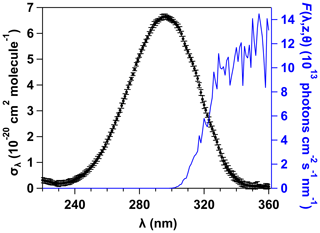Figure 1UV absorption cross sections of 2MP at 298 K. The error bars represent the statistical uncertainty (±2σ).

Photolysis of 2MP follows first-order kinetics, where J is the photolysis rate coefficient to be determined from the slope of ln([2MP]0$/$ [2MP]t) versus time plot (Eq. 1). In Fig. S3 the average values of individual ln([2MP]0$/$ [2MP]t) values obtained in 10 experiments, corrected with kheterog, are plotted against t. Under the experimental irradiation conditions, J (±2σ) was (2.2±0.1) × 10−5 s−1. The effective photolysis quantum yield of 2MP at λ 290 nm, Φeff (2MP), was calculated from Eq. (3) as described in Asensio et al. (2022),

$\begin{array}{}\text{(3)}& J\phantom{\rule{0.125em}{0ex}}\cong {\mathrm{\Phi }}_{\mathrm{eff}}\left(\mathrm{2}\mathrm{MP}\right){\sum }_{\mathrm{290}\phantom{\rule{0.125em}{0ex}}\mathrm{nm}}^{\mathrm{360}\phantom{\rule{0.125em}{0ex}}\mathrm{nm}}{I}_{\mathit{\lambda }}{\mathit{\sigma }}_{\mathit{\lambda }}\mathrm{\Delta }\mathit{\lambda },\end{array}$

where Iλ (in photons cm−2 s−1 nm−1) is the irradiance of the solar simulator at each wavelength, σλ is the UV absorption cross section of 2MP measured in this work, and Δλ= 1 nm. Considering all these parameters, the effective quantum yield of 2MP is Φeff (2MP) = (0.32±0.03). No quantum yields of 2MP have been previously reported. However, for other C-6 aldehydes like hexanal or 3-methylpentanal, the reported Φeff values were similar (0.28±0.05 in Wenger, 2006, and 0.34 in Rebbert and Ausloos, 1967, respectively) to that determined in this work.

The UV photodissociation of 2MP can proceed through the different channels (Reactions R1a–R1d) similarly to other aldehydes (see, e.g., Wenger, 2006). Under atmospheric conditions, the Norrish type I (R1a) and type II (R1c) processes are the most important pathways (Moortgat, 2001). Reactions (R1a) and (R1d) are radical-forming channels, whilst the Norrish type II process and Reaction (R1b) produce stable molecules with no radical formation (pentane and CO for the Reaction R1b and 2-buten-2-ol and propene for the Reaction R1c). Radicals from Reactions (R1a) and (R1d) may generate 2-pentanone and 2-pentanol as primary products of the reaction of CH3CH2CH2CH(CH3) and CH3CH2CH2CH(CH3)CO with O2, respectively:

$\begin{array}{}\text{(R1a)}& \begin{array}{rl}& {\mathrm{CH}}_{\mathrm{3}}{\mathrm{CH}}_{\mathrm{2}}{\mathrm{CH}}_{\mathrm{2}}\mathrm{CH}\left({\mathrm{CH}}_{\mathrm{3}}\right)\mathrm{C}\left(\mathrm{O}\right)\mathrm{H}+h\mathit{\nu }\\ & \to {\mathrm{CH}}_{\mathrm{3}}{\mathrm{CH}}_{\mathrm{2}}{\mathrm{CH}}_{\mathrm{2}}\mathrm{CH}\left({\mathrm{CH}}_{\mathrm{3}}\right)+\mathrm{HCO}\end{array},\text{(R1b)}& \to {\mathrm{CH}}_{\mathrm{3}}{\mathrm{CH}}_{\mathrm{2}}{\mathrm{CH}}_{\mathrm{2}}{\mathrm{CH}}_{\mathrm{2}}{\mathrm{CH}}_{\mathrm{3}}+\mathrm{CO},\text{(R1c)}& \to {\mathrm{CH}}_{\mathrm{3}}\left(\mathrm{OH}\right)\mathrm{C}={\mathrm{CHCH}}_{\mathrm{2}}+{\mathrm{CH}}_{\mathrm{3}}\mathrm{CH}={\mathrm{CH}}_{\mathrm{2}},\text{(R1d)}& \to {\mathrm{CH}}_{\mathrm{3}}{\mathrm{CH}}_{\mathrm{2}}{\mathrm{CH}}_{\mathrm{2}}\mathrm{CH}\left({\mathrm{CH}}_{\mathrm{3}}\right)\mathrm{CO}+\mathrm{H}.\end{array}$

In Fig. 2, the IR spectra recorded before and after 150 min of irradiation are presented. The identified photolysis products were 2-pentanone (bands around 3000–2900 and 1732 cm−1), which indicate that the Norrish type I and R1d channels are open, and CO (characteristic band 2000–2300 cm−1) formed directly via Reaction (R1b) and/or from the fast reaction of HCO radicals with O2. However, it cannot be assured that the co-product of CO, pentane, was formed since the IR bands used for its identification (3000–2800 and 1480–1340 cm−1) can also be attributed to propene. Therefore, the Norrish type II process could also be open. The reference IR spectra used in the identification of these products are shown in Fig. S4. After subtracting the IR features of CO, 2-pentanone, pentane, and propene, some IR features are still in the residual spectrum (see Fig. 2c). The remaining features can be assigned to oxygenated compounds formed from the chemistry of the radicals generated in Reactions (R1a) and (R1d) (for example, 2-pentanol from oxidation of CH3CH2CH2CH(CH3) and CH3CH2CH2CH(CH3)CO radicals) or butanone formed by the keto-enolic tautomerism of 2-buten-2-ol (reaction product of R1c). The quantification of the reaction products identified was very imprecise. For that reason, no molar yield is provided.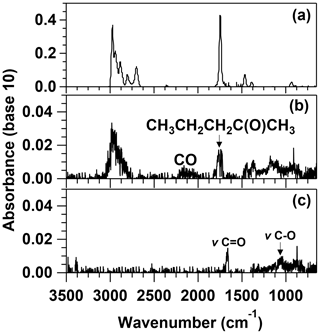Figure 2FTIR spectra of the 2MP$\phantom{\rule{0.125em}{0ex}}/\phantom{\rule{0.125em}{0ex}}$air mixture: (a) before irradiation, (b) after 150 min of photolysis (features of unreacted 2MP were subtracted), and (c) residual spectrum after subtraction of the reference spectra of the identified products (CO, 2-pentanone, pentane, and propene) shown in Fig. S4.

## 3.2 Kinetics and products of the 2MP + Cl reaction

Relative rate plots (Eq. 2) are shown in Fig. S5. The slope of these plots yields the individual ${k}_{\mathrm{Cl}}/{k}_{\mathrm{ref}}$ values listed in Table 1 for each reference compound. The good linearity of such plots suggests that the extent of secondary reactions was negligible. The average rate coefficient kCl, reported here for the first time, is

${k}_{\mathrm{Cl}}=\left(\mathrm{2.2}±\mathrm{0.4}\right)×{\mathrm{10}}^{-\mathrm{10}}\phantom{\rule{0.125em}{0ex}}{\mathrm{cm}}^{\mathrm{3}}\phantom{\rule{0.125em}{0ex}}\mathrm{molec}{.}^{-\mathrm{1}}\phantom{\rule{0.125em}{0ex}}{\mathrm{s}}^{-\mathrm{1}}.$

The stated uncertainty (±2σ) in kCl includes the propagation of the reported errors in kref, the statistical errors from the slope of the plots shown in Fig. S5, and the systematic uncertainties (estimated to be ±10 % of kCl). In the Supplement, a detailed description of the error analysis can be found.

Table 1Summary of relative rate measurements for 2MP at 298±2 K and 760±5 Torr of air. Errors include statistical ±2σ and 10 % systematic uncertainties (see text).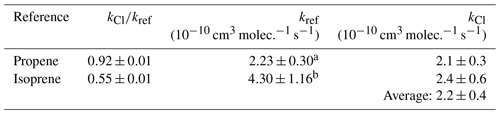a Ceacero-Vega et al. (2009). b Orlando et al. (2003).

The GC-MS technique was only used as an identification technique. Figure S6 shows the chromatogram obtained before (t=0) and after 40 min of irradiation. The following reaction products were identified: acetaldehyde, butanedial, acetic acid, 2-pentanone, 3-pentanone, butanal, and 2-methylbutanoic acid. The mass spectra of 2MP and the identified products are shown in Fig. S7. No products were observed during the UV light exposure of 2MP in the absence of Cl precursor or in its dark reaction with Cl2.

Not all the reaction products identified by GC–MS instrument were detected by FTIR spectroscopy. The products identified by FTIR were CO, HCl, 2-pentanone, acetaldehyde, 2-methylbutanoic acid, acetic acid, and formaldehyde. The IR spectra used for quantification of 2-pentanone, acetic acid, acetaldehyde, formaldehyde, HCl, and CO were recorded in our lab, while those of pentane, 2-methylbutanoic acid, and propene were taken from the NIST FTIR database (Fig. S4). After subtracting the IR features of all these products, some bands between 1900 and 1100 cm−1 still remain in the residual spectrum (see the bottom of Fig. S8). This could be due to the other compounds with lower concentration identified by GC–MS instrument for which the detection by FTIR was difficult due to the lack of characteristic bands in the residual spectrum. The remaining bands could correspond to butanal, butanedial, or a mixture of both. The molar yields for the major products, YProduct, were obtained from the slope of [product] versus consumed [2MP] plots (Fig. S9a). YProduct values (±2σ) were (84.6±3.4) % for HCl, (23.9±0.6) % for 2-pentanone, and (11.1±0.3) % for acetaldehyde.

All reaction products identified by GC–MS instrument and FTIR spectroscopy, except CO, were also observed by PTR-ToF-MS. These products are formaldehyde (CH2OH+, $m/z=$ 31.02), acetaldehyde (C2H4OH+, $m/z=$ 45.03), acetic acid (C2H4O2H+, $m/z=$ 61.02), 2-pentanone (C5H10OH+, $m/z=$ 87.08), 2-methylbutanoic acid (C5H10O2H+, $m/z=$ 103.13), butanedial (C4H6O2H+, $m/z=$ 87.04), and butanal (C4H8OH+, $m/z=$ 73.06). In addition, other products were also observed such as methanol (CH4OH+, $m/z=$ 33.03), methylglyoxal (C3H4O2H+, $m/z=$ 73.03), and propanoic acid (C3H6O2H+, $m/z=$ 75.04) but at lower concentrations. The PTR-ToF-MS signal from the identified products was calibrated to ensure the accuracy in the quantification. From the product yield plots shown in Fig. S9b, YProduct (±2σ) was (18.9±0.4) % for 2-pentanone and (14.1±1.1) % for acetaldehyde.

The time evolution of 2MP and the products identified with an average ion concentration greater than 2×1011 molecule cm−3 (8.1 ppb) is shown in Fig. 3. Although these products were also formed during the 2MP exposure to the UV light in the test experiments (without Cl2) performed prior to the Cl reaction, their concentration was negligible (< 4×1010 molecules cm${}^{-\mathrm{3}}\equiv$ 1.6 ppb) compared with the observed levels after the Cl reaction. For minor products, no yield values are provided.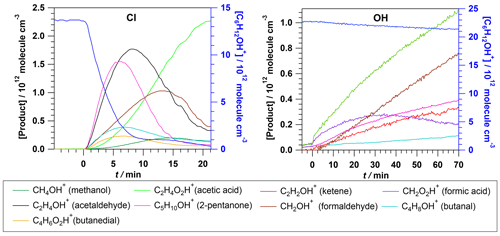Figure 3Time evolution of 2MP (C6H12OH+) and the products measured by PTR-ToF-MS during the Cl and OH reactions.

The presence of HCl as a primary product in Reacton (R2) indicates that the reaction proceeds via H abstraction mainly from one of the following three sites:

$\begin{array}{}\text{(R2a)}& \begin{array}{rl}& {\mathrm{CH}}_{\mathrm{3}}{\mathrm{CH}}_{\mathrm{2}}{\mathrm{CH}}_{\mathrm{2}}\mathrm{CH}\left({\mathrm{CH}}_{\mathrm{3}}\right)\mathrm{C}\left(\mathrm{O}\right)\mathrm{H}+\mathrm{Cl}\\ & \to {\mathrm{CH}}_{\mathrm{3}}{\mathrm{CH}}_{\mathrm{2}}{\mathrm{CH}}_{\mathrm{2}}\mathrm{CH}\left({\mathrm{CH}}_{\mathrm{3}}\right)\mathrm{C}\left(\mathrm{O}\right)+\mathrm{HCl},\end{array}\text{(R2b)}& \to {\mathrm{CH}}_{\mathrm{3}}{\mathrm{CH}}_{\mathrm{2}}{\mathrm{CH}}_{\mathrm{2}}\mathrm{C}\left({\mathrm{CH}}_{\mathrm{3}}\right)\mathrm{C}\left(\mathrm{O}\right)\mathrm{H}+\mathrm{HCl},\text{(R2c)}& \to {\mathrm{CH}}_{\mathrm{3}}{\mathrm{CH}}_{\mathrm{2}}\mathrm{CHCH}\left({\mathrm{CH}}_{\mathrm{3}}\right)\mathrm{C}\left(\mathrm{O}\right)\mathrm{H}+\mathrm{HCl}.\end{array}$

Two of the major products, HCl and acetaldehyde, can be explained by any of the three pathways mentioned above (Reactions R2a–R2c). The first step leads to the formation of HCl and a radical, after several reactions with O2 or RO2 radicals, that forms acetaldehyde. Reactions (R2a) and (R2b) lead to the generation of 2-pentanone, another major product. In Reaction (R2a), the carbonyl radical obtained after the H abstraction from the C(O)H group reacts with O2 and RO2 radicals, after subsequent reactions, yielding 2-pentanone. In addition, the decomposition of the radical prior to the formation of 2-pentanone generates butanal, detected by PTR-ToF-MS and GC–MS instrument. Other minor products, such as formaldehyde and methanol, are generated through the three pathways as final decompositions products. Finally, the decomposition of CH3CH2CH2C(CH3)C(O)H and CH3CH2CHCH(CH3)C(O)H radicals from Reactions (R2b) and (R2c) generates methylglyoxal, another minor product detected by PTR-ToF-MS.

### 3.2.1 Formation of secondary organic aerosols (SOAs)

The size distribution of the particles formed in the Cl + 2MP reaction with mobility diameters (Dp) between 6 and 523 nm is shown in terms of the normalized particle number, dN$/$ dlogDp, and mass, dM$/$ dlogDp, in Fig. 4. As shown in Fig. 4a, after 2–4 min (t= 0 min corresponds to the beginning of Cl reaction), the maximum dN$/$dlogDp corresponds to particles with a diameter of ca. 100–150 nm, while the maximum dM$/$ dlogDp was reached at t= 10 min for particles of ca. 500 nm (Fig. 4b), close to the maximum Dp that can be detected by the FMPS. Due to this instrumental limitation, the total SOA mass formed could not be inferred.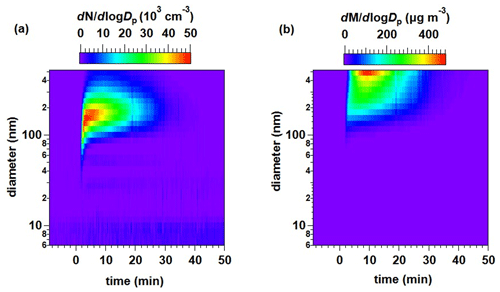Figure 4Time evolution of the size of the SOA generated in the 2MP + Cl reaction in terms of the (a) normalized particle number and (b) mass.

## 3.3 Kinetics and products of the 2MP + OH reaction

Since no pressure dependence of the individual second-order rate coefficient, kOH(T), for the OH + 2MP reaction was found between 50 and 500 Torr, as shown in Table S4, the rate coefficients at a single temperature were obtained by plotting ${k}^{\prime }-{k}_{\mathrm{0}}^{\prime }$ vs. [2MP]0 at different total pressures (Fig. 5). For example, at room temperature,

${k}_{\mathrm{OH}}\left(\mathrm{298}\phantom{\rule{0.125em}{0ex}}\mathrm{K}\right)=\left(\mathrm{3.3}±\mathrm{0.3}\right)×{\mathrm{10}}^{-\mathrm{11}}\phantom{\rule{0.125em}{0ex}}{\mathrm{cm}}^{\mathrm{3}}\phantom{\rule{0.125em}{0ex}}\mathrm{molec}{.}^{-\mathrm{1}}\phantom{\rule{0.125em}{0ex}}{\mathrm{s}}^{-\mathrm{1}},$

which is in excellent agreement with that reported by D'Anna et al. (2001), kOH (298 K) = (3.32±0.14) × 10−11 cm3 molec.−1 s−1. To our knowledge, this is the first kinetic study of Reaction (R3) as a function of temperature. In the studied range of temperature (263–353 K), kOH (T) shows a slightly negative temperature dependence, increasing by around 61 % from 353 to 263 K, as shown in Table S4. The values of kOH (T) fit to the Arrhenius equation (Fig. S10). The Arrhenius parameters found were the pre-exponential factor A= (6.18±0.94) × 10−12 cm3 molec.−1 s−1 and ${E}_{\mathrm{a}}/R=$ (509±45) K, i.e., an activation energy of Ea= $-\left(\mathrm{4.23}±\mathrm{0.38}\right)$ kJ mol−1. Uncertainties in A and Ea correspond to 2σ statistical errors.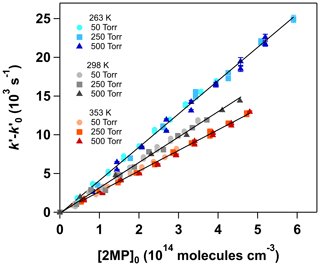Figure 5Examples of the ${k}^{\prime }-{k}_{\mathrm{0}}^{\prime }$ vs. [2MP]0 plots (Eq. S3) at 263, 298 and 353 K in the 50–500 Torr pressure range.

Figure 3 shows the temporal profile of 2MP and the products identified in the OH reaction by PTR-ToF-MS. In the timescale of the experiment, 2MP is less reactive towards OH radicals than towards Cl atoms. The major products were acetaldehyde, formaldehyde, and 2-pentanone with lower concentrations than those generated in the Cl reaction. The reason for such observations can also be related to a low OH concentration generated in the photolysis of H2O2. This suggests that the OH reaction proceeds by a H-abstraction pathway similarly to the Cl reaction. Other minor products were ketene (C2H2OH+, $m/z=$ 43.02), formic acid (CH2O2H+, $m/z=$ 47.01), and butanal. Nevertheless, as confirmed in prior experiments in the absence of OH radicals, acetaldehyde, 2-pentanone, and formaldehyde were also formed at lower concentrations than those observed after the OH reaction, in the dark reaction of 2MP with H2O2 (1.6 × 1011, 3.59 × 1010, and 3.37 × 1010 molecules cm−3 (6, 1, and 1 ppb), respectively), and by UV photolysis (3.76 × 1011, 4.53 × 1010, and 1.01 × 1011 molecules cm−3 (10, 2, and 4 ppb), respectively).

4 Atmospheric implications

Considering the most important degradation pathways (UV photolysis and reactions with Cl atoms and OH radicals), the lifetime of 2MP, τ2MP, can be estimated according to Eq. (4):

$\begin{array}{}\text{(4)}& {\mathit{\tau }}_{\mathrm{2}\mathrm{MP}}=\frac{\mathrm{1}}{J\left(z,\mathit{\theta }\right)+{k}_{\mathrm{Cl}}\left[\mathrm{Cl}\right]+{k}_{\mathrm{OH}}\left[\mathrm{OH}\right]},\end{array}$

where J(z,θ) is the photolysis rate of 2MP at a certain z and θ; kCl and kOH are those determined in this work at 298 K; and [Cl] and [OH] are the concentrations of Cl atoms and OH radicals, depending on the considered scenario (Table 2).

Table 3Estimated lifetimes of 2MP due to the investigated processes.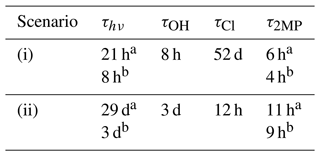a In wintertime. b In summertime.

Table 4Summary of identified reaction products in Reactions (R1), (R2), and (R3) and analytical techniques used.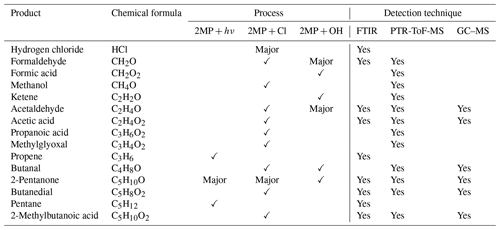In this work, the following scenarios for a mid-latitude urban atmosphere were considered:

• i.

Inland. This is for a winter and a summer day at 13:00 GMT+1 in Ciudad Real (z= 0.6 km).

• ii.

Coastal. This is for a winter and a summer day in Valencia (z= 0 km) at dawn, where the Cl chemistry can play a significant role.

The photolysis rate coefficient J(z,θ) was estimated as follows (Jiménez et al., 2007):

$\begin{array}{}\text{(5)}& J\left(z,\mathit{\theta }\right)\cong {\mathrm{\Phi }}_{\mathrm{eff}}\left(\mathrm{2}\mathrm{MP}\right){\sum }_{\mathit{\lambda }\phantom{\rule{0.125em}{0ex}}>\phantom{\rule{0.125em}{0ex}}\mathrm{290}\phantom{\rule{0.125em}{0ex}}\mathrm{nm}}F\left(\mathit{\lambda },z,\mathit{\theta }\right){\mathit{\sigma }}_{\mathit{\lambda }}\mathrm{\Delta }\mathit{\lambda },\end{array}$

where σλ values used in the calculation were those listed in Table S3, and Φeff= 0.32 was determined in this work. F(λ, z, θ) (in photons cm−2 nm−1 s−1) is the solar spectral actinic flux for a specific θ in the troposphere, obtained using the TUV radiative transfer model (5.3 version) developed by Madronich and Flocke (1999), and Δλ= 1 nm. In Table S5, the photolysis rate coefficients obtained for scenarios (i) and (ii) are summarized, and they were used to calculate the lifetime of 2MP due to this process as τhν=$\mathrm{1}/J\left(z,\mathit{\theta }$) (see Table 3).

Considering the concentration of oxidants provided in Table 2, the estimated lifetime of 2MP due to the reaction with OH radicals (${\mathit{\tau }}_{\mathrm{OH}}=\mathrm{1}/\mathit{\left\{}{k}_{\mathrm{OH}}\left[\mathrm{OH}\right]\mathit{\right\}}$) is 8 h in a mid-latitude inland urban atmosphere and 3 d in a coastal atmosphere at dawn. The lifetime of 2MP due to the Cl reaction (${\mathit{\tau }}_{\mathrm{Cl}}=\mathrm{1}/\mathit{\left\{}{k}_{\mathrm{Cl}}\left[\mathrm{Cl}\right]\mathit{\right\}}$) is 52 d in a mid-latitude inland urban atmosphere but 12 h in a mid-latitude coastal urban atmosphere at dawn. As shown in Table 3, the contribution of each process can be derived considering the individual lifetimes. In a mid-latitude inland urban atmosphere, the dominant degradation pathway for 2MP is the reaction with OH radicals in winter, while UV photolysis is a competing process in summer.

Tropospheric removal of 2MP by NO3 radicals is negligible (${\mathit{\tau }}_{{\mathrm{NO}}_{\mathrm{3}}}=$ 41 h) compared to the diurnal removal (on the order of a few hours). To estimate ${\mathit{\tau }}_{{\mathrm{NO}}_{\mathrm{3}}}$, the rate coefficient for the 2MP + NO3 reaction reported by D'Anna et al. (2001) has been considered. In the estimation, a 24 h average [NO3] is preferred, even though NO3 is photolyzed at daytime. Based on a nighttime average [NO3] of 5 × 108 radicals cm−3 (Shu and Atkinson, 1995), the 24 h average [NO3] considered here is 2.5 × 108 radicals cm−3.

Regarding the impact of 2MP degradation on air quality, the consequences extend beyond the formation of carbonyl compounds mentioned earlier in low-NOx areas. The detected products, as summarized in Table 4, highlight the presence of various VOCs that, in polluted environments, may play a significant role in the generation of photochemical smog. For instance, 2-pentanone, which emerges as the most abundant VOC resulting from the Cl + 2MP reaction and UV photolysis of 2MP, is also formed in the OH+2MP reaction. Additionally, acetaldehyde and formaldehyde, the predominant VOCs in the OH + 2MP reaction, may further contribute to photochemical smog.

As 2MP is primarily emitted to the atmosphere from anthropogenic sources, NOx chemistry typical of polluted environments cannot be fully decoupled. When high levels of NOx are present, ozone is formed. The amount of tropospheric ozone that may be generated from the degradation of 2MP initiated by OH radicals (POCP, photochemical ozone creation potential) is estimated to be 46 (relative to ethene, POCP = 1). This parameter for 2MP and, therefore, its contribution to photochemical smog are on the same order of magnitude as other aldehydes (Jenkin et al., 2017). Moreover, in these environments, it is likely that toxic and irritant peroxyacyl nitrates (PANs) will be formed.

Furthermore, the evidence obtained from this research demonstrates the fast formation and subsequent growth of SOAs. Nonetheless, these findings must be interpreted cautiously, as the concentrations of Cl2 and 2MP employed in the SOA experiments were notably higher in comparison to what is typically expected in the atmosphere.

In polluted sites, such as coastal areas or locations with ceramic industries and coal-fired power plants (Galán et al., 2002; Sarwar and Bhave, 2007; Deng et al., 2014), the photolysis of Cl2 is the dominant daytime Cl atom source together with ClNO2 (see Tham et al., 2016; Liu et al., 2017; Xia et al., 2020). While the Cl+2MP reaction could eventually play a role in the atmospheric HCl budget, given the current 2MP emissions, it is likely to have a relatively minor impact on acid rain compared to other sources of HCl.

5 Conclusions

Once 2MP is emitted, it can be degraded in a few hours during daytime (4–11 h) depending on the location and season. Not only does the direct impact of the degradation of 2MP lead to the formation of harmful carbonyl compounds and the growth of submicron particles, exacerbating photochemical smog, and atmospheric pollution, but it can also contribute to the atmospheric budget of HCl, especially in regions where the concentration of Cl atoms are high, although it will have a small impact on acid rain.

Data availability

Infrared spectra for compounds not measured in this work can be found in the NIST WebBook (https://webbook.nist.gov/chemistry/name-ser/, National Institute of Standards and Technology, 2023).

Supplement

Author contributions

MaríaAs, MaríaAn, and SB conducted the experiments and analyzed the experimental data. EJ and JA designed and supervised the experiments and managed the project. All the co-authors have contributed to preparing the paper and to discussing the obtained results.

Competing interests

The contact author has declared that none of the authors has any competing interests.

Disclaimer

Publisher’s note: Copernicus Publications remains neutral with regard to jurisdictional claims made in the text, published maps, institutional affiliations, or any other geographical representation in this paper. While Copernicus Publications makes every effort to include appropriate place names, the final responsibility lies with the authors.

Acknowledgements

This work has been supported by the regional government of Castilla-La Mancha and the European Regional Development Fund (FEDER) through the CINEMOL project (grant no. SBPLY/19/180501/000052) and by the University of Castilla-La Mancha (UCLM) (grant nos. 2021-GRIN-31279 and 2022-GRIN-34143; cofunded by FEDER). María Asensio and Sergio Blázquez also acknowledge CINEMOL and UCLM (Plan Propio de Investigación), respectively, for funding their contracts during the performance of this investigation.

Financial support

This research has been supported by the regional government of Castilla–La Mancha and the European Regional Development Fund (FEDER) through the CINEMOL project (grant no. SBPLY/19/180501/000052) and the University of Castilla-La Mancha (grant nos. 2021-GRIN-31279 and 2022-GRIN-34143; cofunded by FEDER).

Review statement

This paper was edited by Sergey A. Nizkorodov and reviewed by two anonymous referees.

References

Aisala, H., Sola, J., Hopia, A., Linderborg, K. M., and Sandell, M.: Odor-contributing volatile compounds of wild edible Nordic mushrooms analyzed with HS–SPME–GC–MS and HS–SPME–GC–O/FID, Food Chem., 283, 566–578, https://doi.org/10.1016/j.foodchem.2019.01.053, 2019.

Albaladejo, J., Ballesteros, B., Jiménez, E., Martıìn, P., and Martıìnez, E.: A PLP–LIF kinetic study of the atmospheric reactivity of a series of C4–C7 saturated and unsaturated aliphatic aldehydes with OH, Atmos. Environ., 36, 3231-3239, https://doi.org/10.1016/S1352-2310(02)00323-0, 2002.

Antiñolo, M., González, S., Ballesteros, B., Albaladejo, J., and Jiménez, E.: Laboratory Studies of CHF2CF2CH2OH and CF3CF2CH2OH: UV and IR Absorption Cross Sections and OH Rate Coefficients between 263 and 358 K, J. Phys. Chem. A, 116, 6041–6050, https://doi.org/10.1021/jp2111633, 2012.

Antiñolo, M., del Olmo, R., Bravo, I., Albaladejo, J., and Jiménez, E.: Tropospheric fate of allyl cyanide (CH2= CHCH2CN): Kinetics, reaction products and secondary organic aerosol formation, Atmos. Environ., 219, 117041, https://doi.org/10.1016/j.atmosenv.2019.117041, 2019.

Antiñolo, M., Asensio, M., Albaladejo, J., and Jiménez, E.: Gas-Phase Reaction of trans-2-Methyl-2-butenal with Cl: Kinetics, Gaseous Products, and SOA Formation, Atmosphere, 11, 715, https://doi.org/10.3390/atmos11070715, 2020.

Asensio, M., Antiñolo, M., Blázquez, S., Albaladejo, J., and Jiménez, E.: Evaluation of the daytime tropospheric loss of 2-methylbutanal, Atmos. Chem. Phys., 22, 2689–2701, https://doi.org/10.5194/acp-22-2689-2022, 2022.

Atkinson, R. and Arey, J.: Atmospheric Degradation of Volatile Organic Compounds, Chem. Rev., 103, 4605–4638, https://doi.org/10.1021/cr0206420, 2003.

Atkinson, R., Tuazon, E. C., and Aschmann, S. M.: Products of the Gas-Phase Reactions of a Series of 1-Alkenes and 1-Methylcyclohexene with the OH Radical in the Presence of NO, Environ. Sci. Technol., 29, 1674–1680, https://doi.org/10.1021/es00006a035, 1995.

Ballesteros, B., Jiménez, E., Moreno, A., Soto, A., Antiñolo, M., and Albaladejo, J.: Atmospheric fate of hydrofluoroolefins, CxF2x+1CH = CH2 (x= 1, 2, 3, 4 and 6): Kinetics with Cl atoms and products, Chemosphere, 167, 330–343, https://doi.org/10.1016/j.chemosphere.2016.09.156, 2017.

Bao, M.-l., Pantani, F., Griffini, O., Burrini, D., Santianni, D., and Barbieri, K.: Determination of carbonyl compounds in water by derivatization–solid-phase microextraction and gas chromatographic analysis, J. Chromatogr. A, 809, 75-87, https://doi.org/10.1016/S0021-9673(98)00188-5, 1998.

Blázquez, S., Antiñolo, M., Nielsen, O. J., Albaladejo, J., and Jiménez, E.: Reaction kinetics of (CF3)2CFCN with OH radicals as a function of temperature (278–358 K): a good replacement for greenhouse SF6?, Chem. Phys. Lett., 687, 297–302, https://doi.org/10.1016/j.cplett.2017.09.039, 2017.

Blázquez, S., González, D., Neeman, E. M., Ballesteros, B., Agúndez, M., Canosa, A., Albaladejo, J., Cernicharo, J., and Jiménez, E.: Gas-phase kinetics of CH3CHO with OH radicals between 11.7 and 177.5 K, Phys. Chem. Chem. Phys., 22, 20562–20572, https://doi.org/10.1039/D0CP03203D, 2020.

Calvert, J. G., Mellouki, A., Orlando, J. J., Pilling, M. J., and Wallington, T. J.: The Mechanisms of Atmospheric Oxidation of the Oxygenates, Oxford University Press, 198 Madison Avenue, New York, New York 10016, 1634 pp., ISBN 9780199767076, 2011.

Ceacero-Vega, A. A., Ballesteros, B., Albaladejo, J., Bejan, I., and Barnes, I.: Temperature dependence of the gas-phase reactions of Cl atoms with propene and 1-butene between 285 <T< 313 K, Chem. Phys. Lett., 484, 10–13, https://doi.org/10.1016/j.cplett.2009.10.080, 2009.

Ciccioli, P., Brancaleoni, E., Cecinato, A., Sparapani, R., and Frattoni, M.: Identification and determination of biogenic and anthropogenic volatile organic compounds in forest areas of Northern and Southern Europe and a remote site of the Himalaya region by high-resolution gas chromatography–mass spectrometry, J. Chromatogr. A, 643, 55–69, https://doi.org/10.1016/0021-9673(93)80541-F, 1993.

Clarisse, B., Laurent, A. M., Seta, N., Le Moullec, Y., El Hasnaoui, A., and Momas, I.: Indoor aldehydes: measurement of contamination levels and identification of their determinants in Paris dwellings, Environ. Res., 92, 245–253, https://doi.org/10.1016/S0013-9351(03)00039-2, 2003.

D'Anna, B., Andresen, Ø., Gefen, Z., and Nielsen, C. J.: Kinetic study of OH and NO3 radical reactions with 14 aliphatic aldehydes, Phys. Chem. Chem. Phys., 3, 3057–3063, https://doi.org/10.1039/B103623H, 2001.

Deng, S., Zhang, C., Liu, Y., Cao, Q., Xu, Y.-Y., Wang, H.-L., and Zhang, F.: A Full-Scale Field Study on Chlorine Emission of Pulverized Coal-Fired Power Plants in China, Res. Environ. Sci., 27, 127, https://doi.org/10.13198/j.issn.1001-6929.2014.02.03, 2014.

Furia, T. E. and Bellanca, N.: Fenaroli's handbook of flavor ingredients, Vol. 2, Ed 2., CRC Press, Inc., ISBN 0878195327, 1975.

Galán, E., González, I., and Fabbri, B.: Estimation of fluorine and chlorine emissions from Spanish structural ceramic industries. The case study of the Bailén area, Southern Spain, Atmos. Environ., 36, 5289–5298, https://doi.org/10.1016/S1352-2310(02)00645-3, 2002.

Gueymard, C. A., Myers, D., and Emery, K.: Proposed reference irradiance spectra for solar energy systems testing, Sol. Energy, 73, 443–467, https://doi.org/10.1016/S0038-092X(03)00005-7, 2002.

Holland, F., Hofzumahaus, A., Schäfer, J., Kraus, A., and Pätz, H.-W.: Measurements of OH and HO2 radical concentrations and photolysis frequencies during BERLIOZ, J. Geophys. Res.-Atmos., 108, PHO 2-1–PHO 2-23, https://doi.org/10.1029/2001JD001393, 2003.

Jenkin, M. E., Derwent, R. G., and Wallington, T. J.: Photochemical ozone creation potentials for volatile organic compounds: Rationalization and estimation, Atmos. Environ., 163, 128–137, https://doi.org/10.1016/j.atmosenv.2017.05.024, 2017.

Jiménez, E., Lanza, B., Garzón, A., Ballesteros, B., and Albaladejo, J.: Atmospheric degradation of 2-butanol, 2-methyl-2-butanol, and 2,3-dimethyl-2-butanol: OH kinetics and UV absorption cross sections, J. Phys. Chem. A, 109, 10903–10909, https://doi.org/10.1021/jp054094g, 2005.

Jiménez, E., Lanza, B., Martínez, E., and Albaladejo, J.: Daytime tropospheric loss of hexanal and trans-2-hexenal: OH kinetics and UV photolysis, Atmos. Chem. Phys., 7, 1565–1574, https://doi.org/10.5194/acp-7-1565-2007, 2007.

Lippmann, M.: Environmental toxicants: human exposures and their health effects, John Wiley & Sons, ISBN 0471292982, 2000.

Liu, X., Qu, H., Huey, L. G., Wang, Y., Sjostedt, S., Zeng, L., Lu, K., Wu, Y., Hu, M., Shao, M., Zhu, T., and Zhang, Y.: High Levels of Daytime Molecular Chlorine and Nitryl Chloride at a Rural Site on the North China Plain, Environ. Sci. Technol., 51, 9588–9595, https://doi.org/10.1021/acs.est.7b03039, 2017.

National Institute of Standards and Technology: NIST Chemistry WebBook, SRD 69, https://webbook.nist.gov/chemistry/name-ser/, last access: 8 November 2023.

Madronich, S. and Flocke, S.: The Role of Solar Radiation in Atmospheric Chemistry, in: Environmental Photochemistry, edited by: Boule, P., Springer Berlin Heidelberg, Berlin, Heidelberg, 1–26, https://doi.org/10.1007/978-3-540-69044-3_1, 1999.

Martínez, E., Albaladejo, J., Jiménez, E., Notario, A., and Aranda, A.: Kinetics of the reaction of CH3S with NO2 as a function of temperature, Chem. Phys. Lett., 308, 37–44, https://doi.org/10.1016/S0009-2614(99)00579-5, 1999.

Moortgat, G. K.: Important photochemical processes in the atmosphere, Pure Appl. Chem., 73, 487–490, https://doi.org/10.1351/pac200173030487, 2001.

Orlando, J. J., Tyndall, G. S., Apel, E. C., Riemer, D. D., and Paulson, S. E.: Rate coefficients and mechanisms of the reaction of cl-atoms with a series of unsaturated hydrocarbons under atmospheric conditions, Int. J. Chem. Kinet., 35, 334–353, https://doi.org/10.1002/kin.10135, 2003.

Prinn, R. G., Huang, J., Weiss, R. F., Cunnold, D. M., Fraser, P. J., Simmonds, P. G., McCulloch, A., Harth, C., Salameh, P., O'Doherty, S., Wang, R. H. J., Porter, L., and Miller, B. R.: Evidence for Substantial Variations of Atmospheric Hydroxyl Radicals in the Past Two Decades, Science, 292, 1882–1888, https://doi.org/10.1126/science.1058673, 2001.

Rebbert, R. E. and Ausloos, P.: Comparison of the Direct and Sensitized Photolysis of 3-Methylpentanal in the Vapor Phase, J. Am. Chem. Soc., 89, 1573–1579, https://doi.org/10.1021/ja00983a006, 1967.

Rodríguez, A., Rodríguez, D., Soto, A., Bravo, I., Diaz-de-Mera, Y., Notario, A., and Aranda, A.: Products and mechanism of the reaction of Cl atoms with unsaturated alcohols, Atmos. Environ., 50, 214–224, https://doi.org/10.1016/j.atmosenv.2011.12.030, 2012.

Sarwar, G. and Bhave, P. V.: Modeling the Effect of Chlorine Emissions on Ozone Levels over the Eastern United States, J. Appl. Meteorol. Clim., 46, 1009–1019, https://doi.org/10.1175/JAM2519.1, 2007.

Shu, Y. and Atkinson, R.: Atmospheric lifetimes and fates of a series of sesquiterpenes, J. Geophys. Res.-Atmos., 100, 7275–7281, https://doi.org/10.1029/95JD00368, 1995.

Singh, H. B., Thakur, A. N., Chen, Y. E., and Kanakidou, M.: Tetrachloroethylene as an indicator of low Cl atom concentrations in the troposphere, Geophys. Res. Lett., 23, 1529–1532, https://doi.org/10.1029/96GL01368, 1996.

Spicer, C. W., Chapman, E. G., Finlayson-Pitts, B. J., Plastridge, R. A., Hubbe, J. M., Fast, J. D., and Berkowitz, C. M.: Unexpectedly high concentrations of molecular chlorine in coastal air, Nature, 394, 353–356, https://doi.org/10.1038/28584, 1998.

Tham, Y. J., Wang, Z., Li, Q., Yun, H., Wang, W., Wang, X., Xue, L., Lu, K., Ma, N., Bohn, B., Li, X., Kecorius, S., Größ, J., Shao, M., Wiedensohler, A., Zhang, Y., and Wang, T.: Significant concentrations of nitryl chloride sustained in the morning: investigations of the causes and impacts on ozone production in a polluted region of northern China, Atmos. Chem. Phys., 16, 14959–14977, https://doi.org/10.5194/acp-16-14959-2016, 2016.

Villanueva, F., Lara, S., Notario, A., Amo-Salas, M., and Cabañas, B.: Formaldehyde, acrolein and other carbonyls in dwellings of university students. Levels and source characterization, Chemosphere, 288, 132429, https://doi.org/10.1016/j.chemosphere.2021.132429, 2022.

Wenger, J. C.: Chamber Studies on the Photolysis of Aldehydes Environmental, Dordrecht, 111–119, https://doi.org/10.1007/1-4020-4232-9_8, 2006.

Wennberg, P. O., Bates, K. H., Crounse, J. D., Dodson, L. G., McVay, R. C., Mertens, L. A., Nguyen, T. B., Praske, E., Schwantes, R. H., Smarte, M. D., St Clair, J. M., Teng, A. P., Zhang, X., and Seinfeld, J. H.: Gas-Phase Reactions of Isoprene and Its Major Oxidation Products, Chem. Rev., 118, 3337–3390, https://doi.org/10.1021/acs.chemrev.7b00439, 2018.

Xia, M., Peng, X., Wang, W., Yu, C., Sun, P., Li, Y., Liu, Y., Xu, Z., Wang, Z., Xu, Z., Nie, W., Ding, A., and Wang, T.: Significant production of ClNO2 and possible source of Cl2 from N2O5 uptake at a suburban site in eastern China, Atmos. Chem. Phys., 20, 6147–6158, https://doi.org/10.5194/acp-20-6147-2020, 2020.

Xu, X., Stee, L. L. P., Williams, J., Beens, J., Adahchour, M., Vreuls, R. J. J., Brinkman, U. A., and Lelieveld, J.: Comprehensive two-dimensional gas chromatography (GC × GC) measurements of volatile organic compounds in the atmosphere, Atmos. Chem. Phys., 3, 665–682, https://doi.org/10.5194/acp-3-665-2003, 2003.